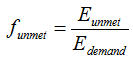# HOMER Pro 3.13

 Navigation: Glossary Unmet Load FractionType: Output Variable Units: none Symbol: funmet

The unmet load fraction is the proportion of the total annual electrical load that went unserved because of insufficient generation. The equation for the unmet load fraction is given below:where: Eunmet = total unmet load [kWh/yr] Edemand = total annual electrical demand (primary plus deferrable) [kWh/yr]